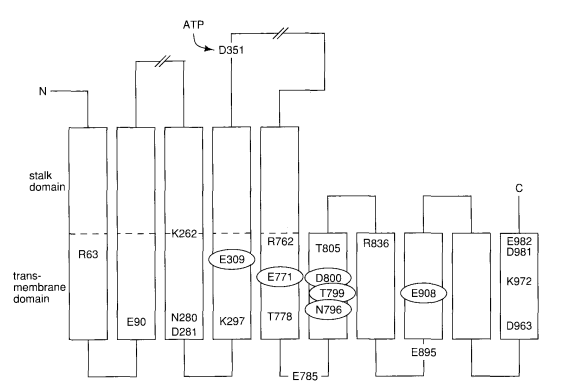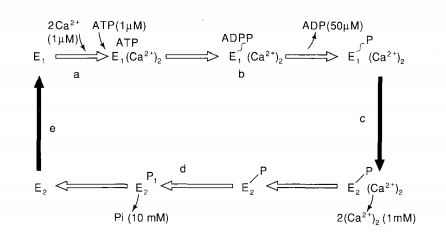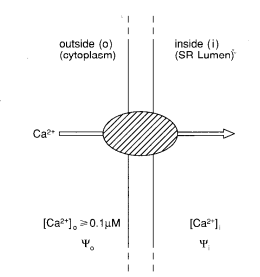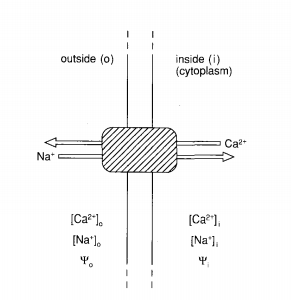# 3.7: Intracellular Calcium Ion Transport

$$\newcommand{\vecs}{\overset { \rightharpoonup} {\mathbf{#1}} }$$ $$\newcommand{\vecd}{\overset{-\!-\!\rightharpoonup}{\vphantom{a}\smash {#1}}}$$$$\newcommand{\id}{\mathrm{id}}$$ $$\newcommand{\Span}{\mathrm{span}}$$ $$\newcommand{\kernel}{\mathrm{null}\,}$$ $$\newcommand{\range}{\mathrm{range}\,}$$ $$\newcommand{\RealPart}{\mathrm{Re}}$$ $$\newcommand{\ImaginaryPart}{\mathrm{Im}}$$ $$\newcommand{\Argument}{\mathrm{Arg}}$$ $$\newcommand{\norm}{\| #1 \|}$$ $$\newcommand{\inner}{\langle #1, #2 \rangle}$$ $$\newcommand{\Span}{\mathrm{span}}$$ $$\newcommand{\id}{\mathrm{id}}$$ $$\newcommand{\Span}{\mathrm{span}}$$ $$\newcommand{\kernel}{\mathrm{null}\,}$$ $$\newcommand{\range}{\mathrm{range}\,}$$ $$\newcommand{\RealPart}{\mathrm{Re}}$$ $$\newcommand{\ImaginaryPart}{\mathrm{Im}}$$ $$\newcommand{\Argument}{\mathrm{Arg}}$$ $$\newcommand{\norm}{\| #1 \|}$$ $$\newcommand{\inner}{\langle #1, #2 \rangle}$$ $$\newcommand{\Span}{\mathrm{span}}$$$$\newcommand{\AA}{\unicode[.8,0]{x212B}}$$

In order to provide a better understanding of the role of Ca2+ as an almost universal regulator of cellular function, we need to take a brief look at the many ways by which Ca2+ ions can be transported in or out of eukaryotic cells. Although various transport pathways have been elucidated, the present picture is probably not complete, since the molecular structures and properties of the transport proteins are only partially known. The major pathways for Ca 2+ transport across cellular membranes involve three membrane systems: the plasma membrane, the inner mitochondrial membrane, and the membrane of the endoplasmic reticulum (ER) (or, in striated muscle cells, a specialized form of ER called the sarcoplasmic reticulum (SR): (Figure 3.9). Two of the membrane-bound transport systems are Ca2+-ATPases, since they derive their main energy from the hydrolysis of ATP (1 and 2 in Figure 3.9). Their properties do, however, differ in many other respects, as we will see.Figure 3.9 - Schematic representation of the major pathways for the transport of Ca2+ across cellular membranes. PM, plasma membrane; ER(SR), endoplasmic reticulum (sarcoplasmic reticulum); M, mitochondria; $$\Delta \Psi$$, difference in membrane potential. The transport proteins shown are: 1 and 2, PM and ER(SR) Ca2+-ATPases; 3 and 4, PM and ER(SR) receptor-mediated Ca2+ channels; 5 and 6, PM and M (inner-membrane) Na+/Ca2+ exchangers; 7 and 8, PM and M voltage-sensitive Ca2+ channels. In addition, some not-well-defined "passive" transport pathways are indicated by dashed arrows.

## The Ca2+-ATPases

The plasma membrane Ca2+-ATPase (PM Ca2+-ATPase) of erythrocytes—first recognized by Schatzmann in 196645—was isolated in pure form by Niggli et al. in 1979, using an affinity column with an ATP-ase binding protein, calmodulin (see Section V.A), coupled to the gel.46 Ca2+-ATPases purified from other types of plasma membranes appear to be very similar. The schematic structure of the erythrocyte membrane Ca2+-ATPase is presented in Figure 3.10.47 The sarcroplasmic reticulum in muscle cells is abundant in Ca2+- ATPase. It is estimated that this protein constitutes more than 80 percent of the integral membrane proteins, and covers a third of the surface area.

The sarcoplasmic reticulum Ca2+-ATPase (SR Ca2+-ATPase) was first purified by MacLennan in 1970.48 Presently it is the best characterized Ca2+-ATPase. A schematic model and a summary of some properties are given in Figure 3.11.49 Ten hydrophobic segments of about 20 amino-acid residues each are revealed by hydropathy plots, and these segments are assumed to span the membrane as $$\alpha$$-helices. (For the one-letter codes for amino acids, see Appendix B in Section IX.) The phosphorylation site has been identified as Asp-351, and the nucleotide binding domain is following the phosphorylation domain. The Ca2+-binding sites are located within the predicted trans-membrane domains (see Figure 3.11). This was shown through a series of site-directed mutations in which likely Ca2+-liganding residues like Asp, GIu, and Thr were mutated into residues lacking possible side-chain ligands (e.g., Asn, GIn, and Ala).50Figure 3.11 - Schematic structure of the Ca2+-ATPase of sarcoplasmic reticulum. Some molecular characteristics are: Mr = 110,000; Km < 1 $$\mu$$M (two Ca2+ sites on cytoplasmic side in high-affinity form); Ca2+/ATP ratio, 2; Mg2+ required for activity. The amino-acid residues labeled were mutated to a residue lacking side chains capable of binding Ca2+. Mutations at the circled positions resulted in complete loss of Ca2+ transport activity, suggesting that the circled residues participate in Ca2+ binding. Adapted from Reference 50.

The presently accepted reaction cycle involves two main alternative conformations, E1 and E2, the former with two high-affinity sites (Km ≲ 1 $$\mu$$M)4 on the cytoplasmic side, which in E2 are open to the luminal side with Km ~ 1 mM.49,51 The mechanism suggested for Ca2+ transport (Figure 3.12) has many features similar to that suggested by Williams for H+ translocation in the mitochondrial ATPase.52Figure 3.12 - Simplified schematic reaction cycle of the Ca2+-ATPase of sarcoplasmic reticulum (SR).49,51 The transport protein is assumed to be in either of two states, E1 on the cytoplasmic side, or E2 on the side of the SR lumen. Starting from E1 in the upper left comer, the reactions steps shown are: (a) binding of Ca2+ and ATP (approximate dissociation constants within parentheses); (b) rapid phosphorylation of Asp-351 of the protein (E1-P) and release of ADP; (c) transformation from an energy-rich, high-Ca2+-affinity conformation (E1-P) (Ca2+)2 to a low-energy, low-affinity conformation (E2-P)(Ca2+)2; (d) hydrolysis of the phosphorylated protein and release of the phosphate into the lumen; (e) return to the original state.

It is instructive to consider briefly the thermodynamic limits of the transport. (The discussions about the thermodynamics behind Ca2+/Na+ transport pertain to Na+/K+ gradients in excitable tissues as well). Let us define an "inside" and an "outside" separated by a membrane, as shown in Figure 3.13, where [Ca2+] and $$\psi$$ denote activities and membrane potentials, respectively. The difference in electrochemical potential, $$\Delta \mu$$, across the membrane for a Ca2+ ion is given by

$\Delta \mu_{Ca^{2+}} = + RT ln\frac{[Ca^{2+}]_{o}}{[Ca^{2+}]_{i}} + 2F \Delta \psi \tag{3.4}$

where F is Faraday's constant, T the temperature, and R the gas constant. If we assume $$\Delta \Psi$$ = 0, which appears reasonable for the SR membrane according to experimental evidence, we may calculate the free-energy change, $$\Delta$$G, at 25 °C for transferring $$\Delta$$n moles of Ca2+ across the membrane. This becomes $$\Delta$$G = -$$\Delta$$n x $$\Delta \mu$$Ca2+ = $$\Delta$$n x 4.1 kcal/mol if [Ca2+]o/[Ca2+]j= 10-3 and $$\Delta$$G = $$\Delta$$n x 5.4 kcal/mol if [Ca2+]o/[Ca2+]j= 10-4. Under the pertinent cellular conditions, the free-energy change associated with ATP hydrolysis to ADP and Pi has been calculated by Tanford to be $$\Delta$$G = -13 to -14 kcal/mol.53 In the absence of a membrane potential, it is thus possible to transport two Ca2+ ions for every ATP molecule hydrolyzed against a concentration (or activity) gradient of 104 or more. This treatment says nothing, of course, about the molecular details of this transport. A more detailed model for the transport cycle has been proposed by Tanford.53Figure 3.13 - Schematic representation of Ca2+ transport through a membrane by a Ca2+-ATPase molecule. $$\Psi$$ denotes membrane potentials.

In the specialized cells of muscle tissue, the sarcoplasmic reticulum may contain much calcium, and if all were "free" Ca2+, the concentration could be as high as 30 mM.54 This value would cause an osmotic pressure difference across the membrane, as well as put a high demand on the SR Ca2+-ATPase. A lowering of the free Ca2+ concentration inside the SR would clearly be beneficial. In the presence of oxalate or phosphate ions in the external medium, calcium oxalate or phosphate may precipitate inside the sarcoplasmic reticulum, but under normal circumstances it appears that Ca2+ ions inside the SR are bound to a very acidic protein, calsequestrin.54 Each molecule (Mr ≈ 40 kDa) is able to bind 40 to 50 Ca2+ ions with an effective dissociation constant of about 1 mM (at I = 0.1). The protein has a low cation specificity and behaves in many respects like a negatively charged polyelectrolyte. It has been crystallized55 and we may soon have access to its x-ray structure.

## The Na+/Ca2+ Exchanger of the Plasma Membrane

Presently available information on the Na+/Ca2+ exchanger has mainly been obtained from studies of the large cells of the giant squid axon and of plasma-membrane vesicles from various other tissues.56,57 In heart plasma-membrane vesicles, the exchanger has the following characteristics: Km = 1.5-5 $$\mu$$M for Ca2+ and ~20 nM for Na+; Vmax ≈ 20 nmol Ca2+/mg protein.58 The stoichiometry is at least 3:1 Na+/Ca2+. Very few molecular details of the exchanger are available at present. We may again briefly consider the thermodynamic framework for an Na+/Ca2+ exchanger (Figure 3. 14). The difference in electrochemical potential for Na and Ca2+ across the membrane is:

$\Delta \mu_{Ca^{2+}} = RT ln\frac{[Ca^{2+}]_{o}}{[Ca^{2+}]_{i}} + 2F \Delta \Psi, \tag{3.5}$

$\Delta \mu_{Na^{+}} = RT ln\frac{[Na^{+}]_{o}}{[Na^{+}]_{i}} + F \Delta \Psi \ldotp \tag{3.6}$Figure 3.14 - Schematic representation of the Ca2+/Na+ exchanger of the plasma membrane. $$\Psi$$ denotes membrane potentials.

The free-energy change, $$\Delta$$GtCa2+, associated with a transfer of $$\Delta$$nCa2+ moles of Ca2+ from the inside to the outside is $$\Delta$$GtCa2+ = $$\Delta$$nCa2+ x $$\Delta \mu$$Ca2+, and the corresponding change associated with the movement of $$\Delta$$nNa+ moles of Na+ from the outside in is $$\Delta$$GtNa+ = - $$\Delta$$nNa+ x $$\Delta \mu$$Na+. If these free-energy changes are coupled via the exchanger, there will be a net flux of Ca2+ as long as the free-energy difference,

$\Delta \Delta G = \Delta G_{t}^{Ca^{2+}} - \Delta G_{t}^{Na^{+}} = \Delta n_{Ca^{2+}} \times \Delta \mu_{Ca^{2+}} - \Delta n_{Na^{+}} \times \Delta \mu_{Na^{+}}, \tag{3.7}$

is less than zero. We can write $$\Delta \Delta$$G for the transport of 1 mol Ca2+ as

$\Delta \Delta G = 2.303 RT \bigg[ log\frac{[Ca^{2+}]_{o}}{[Ca^{2+}]_{i}} - \Delta n_{Na^{+}} \times log\frac{[Na^{+}]_{o}}{[Na^{+}]_{i}} \bigg] + (2 - \Delta n_{Na^{+}}) \times F \Delta \Psi \ldotp \tag{3.8}$

Equating ion activities with concentrations, we note that in a typical mammalian cell [Na+]o ≈ 110-145 mM, and [Na+]i ≈ 7-15 mM, or [Na+]o/[Na+]i ≈ 10. In the absence of a membrane potential difference ($$\Delta \Psi$$ = 0), Equation (3.8) can thus be simplified to

$\Delta \Delta G = 2.3 RT \bigg[ log\frac{[Ca^{2+}]_{o}}{[Ca^{2+}]_{i}} - \Delta n_{Na^{+}} \bigg] \ldotp \tag{3.9}$

To pump one Ca2+ ion out of a cell against a concentration gradient of about 103 (1 $$\mu$$M → 1 mM) requires that at least 3 Na+ ions pass in the opposite direction, thus maintaining $$\Delta \Delta$$G < O. What then will be the effect of a membrane potential difference? Most animal cells, particularly excitable cells such as nerve and muscle cells, have resting potential differences, $$\Delta \Psi$$, over the plasma membrane of 30 to 90 mV (cytoplasm negative). For this value we find the change in free energy, $$\Delta \Delta$$G, for the transport of one mol Ca2+ to be

$\Delta \Delta G = 2.3 RT \bigg[ log\frac{[Ca^{2+}]_{o}}{[Ca^{2+}]_{i}} - \Delta n_{Na^{+}} \bigg] + (2 - \Delta n_{Na^{+}}) 0.1 F \ldotp \tag{3.10}$

Thus for $$\Delta$$nNa+ > 2, we have $$\Delta \Delta$$G < 0, and the transport of Ca2+ against a concentration gradient of about 103 will be promoted. This is another good reason for having a Na+/Ca2+ exchange stoichiometry of 3:1.

3.7: Intracellular Calcium Ion Transport is shared under a CC BY-NC-SA 4.0 license and was authored, remixed, and/or curated by LibreTexts.